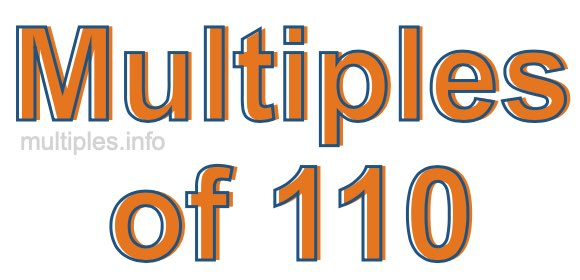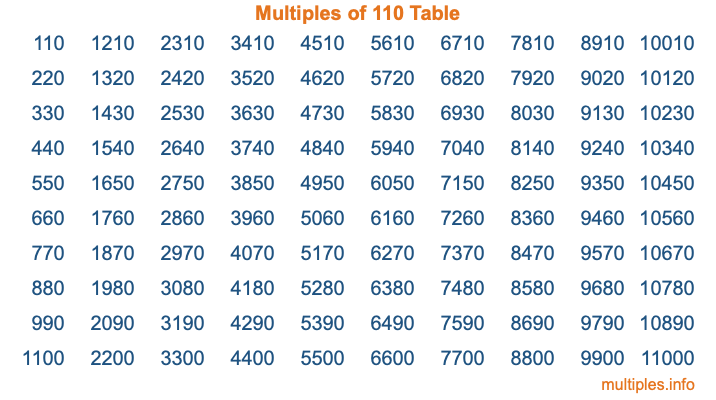Multiples of 110Welcome to the Multiples of 110 page. Here we will first teach you everything you will ever need to know about the multiples of 110, and then give you a study guide summary of everything we taught you to make sure you remember it all. Use this page to look up facts and learn information about the multiples of 110. This page will make you a multiples of one hundred ten expert!

Definition of Multiples of 110
Multiples of 110 are all the numbers that when divided by 110 equal an integer. Each of the multiples of 110 are called a multiple. A multiple of 110 is created by multiplying 110 by an integer.

Therefore, to create a list of multiples of 110, you start with 1 multiplied by 110, then 2 multiplied by 110, then 3 multiplied by 110, and so on for as long as you want. Thus, the list of the first five multiples of 110 is 110, 220, 330, 440, and 550. To see a larger list of multiples of 110, see the printable image of Multiples of 110 further down on this page. We also have a category where you can choose any nth multiple of 110.

Multiples of 110 Checker
The Multiples of 110 Checker below checks to see if any number of your choice is a multiple of 110. In other words, it checks to see if there is any number (integer) that when multiplied by 110 will equal your number. To do that, we divide your number by 110. If the the quotient is an integer, then your number is a multiple of 110.

Is  a multiple of 110?

Least Common Multiple of 110 and ...
A Least Common Multiple (LCM) is the lowest multiple that two or more numbers have in common. This is also called the smallest common multiple or lowest common multiple and is useful to know when you are adding our subtracting fractions. Enter one or more numbers below (110 is already entered) to find the LCM.

Check out our LCM Calculator if you need more details about the Least Common Multiple or if you need the LCM for different numbers for adding and subtraction fractions.

nth Multiple of 110
As we stated above, 110 is the first multiple of 110, 220 is the second multiple of 110, 330 is the third multiple of 110, and so on. Enter a number below to find the nth multiple of 110.

th multiple of 110

Multiples of 110 vs Factors of 110
110 is a multiple of 110 and a factor of 110, but that is where the similarities end. All postive multiples of 110 are 110 or greater than 110. All positive factors of 110 are 110 or less than 110.

Below is the beginning list of multiples of 110 and the factors of 110 so you can compare:

Multiples of 110: 110, 220, 330, 440, 550, etc.

Factors of 110: 1, 2, 5, 10, 11, 22, 55, 110

As you can see, the multiples of 110 are all the numbers that you can divide by 110 to get a whole number. The factors of 110, on the other hand, are all the whole numbers that you can multiply by another whole number to get 110.

It's also interesting to note that if a number (x) is a factor of 110, then 110 will also be a multiple of that number (x).

Multiples of 110 vs Divisors of 110
The divisors of 110 are all the integers that 110 can be divided by evenly. Below is a list of the divisors of 110.

Divisors of 110: 1, 2, 5, 10, 11, 22, 55, 110

The interesting thing to note here is that if you take any multiple of 110 and divide it by a divisor of 110, you will see that the quotient is an integer.

Multiples of 110 Table
Below is an image of the first 100 multiples of 110 in a table. The table is in chronological order, column by column. The first column has the first ten multiples of 110, the second column has the next ten multiples of 110, and so on.The Multiples of 110 Table is also referred to as the 110 Times Table or Times Table of 110. You are welcome to print out our table for your studies.

Negative Multiples of 110
Although not often discussed or needed in math, it is worth mentioning that you can make a list of negative multiples of 110 by multiplying 110 by -1, then by -2, then by -3, and so on, to get the following list of negative multiples of 110:

-110, -220, -330, -440, -550, etc.

Multiples of 110 Summary
Below is a summary of important Multiples of 110 facts that we have discussed on this page. To retain the knowledge on this page, we recommend that you read through the summary and explain to yourself or a study partner why they hold true.

There are an infinite number of multiples of 110.

A multiple of 110 divided by 110 will equal a whole number.

110 divided by a factor of 110 equals a divisor of 110.

The nth multiple of 110 is n times 110.

The largest factor of 110 is equal to the first positive multiple of 110.

110 is a multiple of every factor of 110.

110 is a multiple of 110.

A multiple of 110 divided by a divisor of 110 equals an integer.

110 divided by a divisor of 110 equals a factor of 110.

Any integer times 110 will equal a multiple of 110.

Multiples of a Number
Here you can get the multiples of another number, all with the same attention to detail as we did for multiples of 110 on this page.

Multiples of
Multiples of 111
Did you find our page about multiples of one hundred ten educational? Do you want more knowledge? Check out the multiples of the next number on our list!